Maths-
General
Easy

Question

# Total number of divisors of 480, that are of the form 4n + 2, n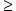0, is equal to :

## 2    3    4    None of theseHint:

## The correct answer is: 4

### Detailed SolutionIn this question, we have been asked to find the total number of divisors of 480 which are of the form  4n + 2, n0 In order to solve this question, we should know that the number of the divisor of any number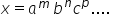where a, b, c are prime numbers and is given by (m + 1) (n + 1) (p + 1)…..We know that 480 can be expressed as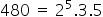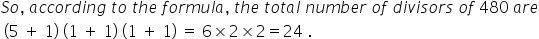Now, we have been asked to find the number of divisors which are of the form 4n + 2 = 2 (2n + 1), which means odd divisors cannot be a part of the solution.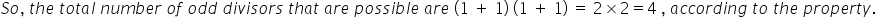Now, we can say the total number of even divisors are = all divisors – odd divisor = 24 - 4 = 20Now, we have been given that the divisor should be of 4n + 2, which means they should not be a multiple of 4 but multiple of 2. For that, we will subtract the multiple of 4 which are divisor of 480 from the even divisors.And, we know that,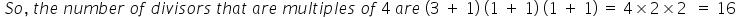Hence, we can say that there are 16 divisors of 480 which are multiple of 4.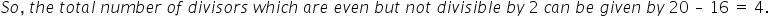Thus, total number of divisors of 480, that are of the form 4n + 2, n0, is equal to 4.

We can also solve this question by writing 4n + 2 = 2(2n + 1) where 2n + 1 is always an odd number. So, when all odd divisors will be multiplied by 2, we will get the divisors that we require. Hence, we can say a number of divisors of 4n + 2 form is the same as the number of odd divisors for 480.#### With Turito Foundation.#### Get an Expert Advice From Turito.